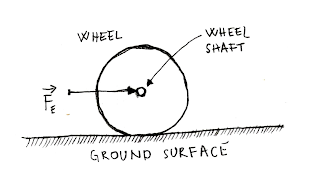### Feel the force around you – Frictional force Part IV

This is the fourth article in a series in order to explain the difference between sliding and rolling motion. There is an obvious difference between these two motions and it will be explained in this blog post, article as part of frictional force articles.

To explain the difference between these two motions we will use a wheel and ground surface presented on figure number one (Figure 1).Figure 1. Wheel and ground surface

Sliding motion (Figure 2) always exists if the frictional force does not exist or it has very small intensity. If the frictional force does not exist at all, with zero intensity, that means that one of the surfaces is perfectly smooth (in theory), let’s assume that it is a ground in this case. If an external force is applied at the wheel shaft, sliding will be provided as the final result, which means that rolling/rotation does not exist. In this case only applied external force exist, and it is not resisted by frictional force, because it is zero in intensity. Rolling motion exists only if both surfaces are rough.Figure 2. Sliding motion, ground surface is perfectly smooth.

Let’s explain second example (Figure 3), with ground surface which has roughness. Now, if external force is applied to wheel shaft, little roughness presented on the ground surface will act in opposite directions, as a resistor to external force, providing frictional force in the opposite direction, forcing the wheel to roll in forward direction. But that is not all. At this point we do have two different scenarios. If there is no relative motion between wheel and a ground, rolling motion will be continued in the forward direction, at the same time providing torque on the wheel shaft (In mobile robotics, wheel torque is provided/generated by DC motors). Because relative motion does not exist between the wheel and ground surface, that means that rolling motion is stable, and it is generated by static friction. Otherwise, if the relative motion exists between wheel and ground surfaces, rolling motion becomes spinning motion. In that case, static friction does not exist between surfaces. Instead, kinetic friction acts between wheel and ground surface providing spinning. Only static friction provides controlled rolling motion, while kinetic friction provides spinning motion. If you are Formula 1 fun, Traction Control System is very well known to you, because it controls wheel to stay under static friction domain at the moment of Formula 1 car startup, not allowing the wheel to spin around, for example.Figure 3. Rolling motion does exist, with generated torque at wheel shaft.

As we already have had discussion, depending on geometric shape of the object, static friction in some cases provides motion, for rounded objects like a wheel and does not provide the motion for cubical shaped objects.

Related articles:
Feel the force around you – Normal force
Normal force - Real catalog examples and calculations
Feel the force around you – Frictional force Part I
Feel the force around you – Frictional force Part II
Feel the force around you – Frictional force Part III